# 使用XcodePython进行IOS运动轨迹模拟## 硬件环境：

MacBook Pro (15-inch, 2017)

iPhone 8 Plus

## 软件环境：

macOS Mojave 10.14.5

iOS 12.4

Xcode 10.2.1

Python 3.7.0

sublime text 3.1.1

# 具体步骤

## 1.使用Xcode创建一个IOS项目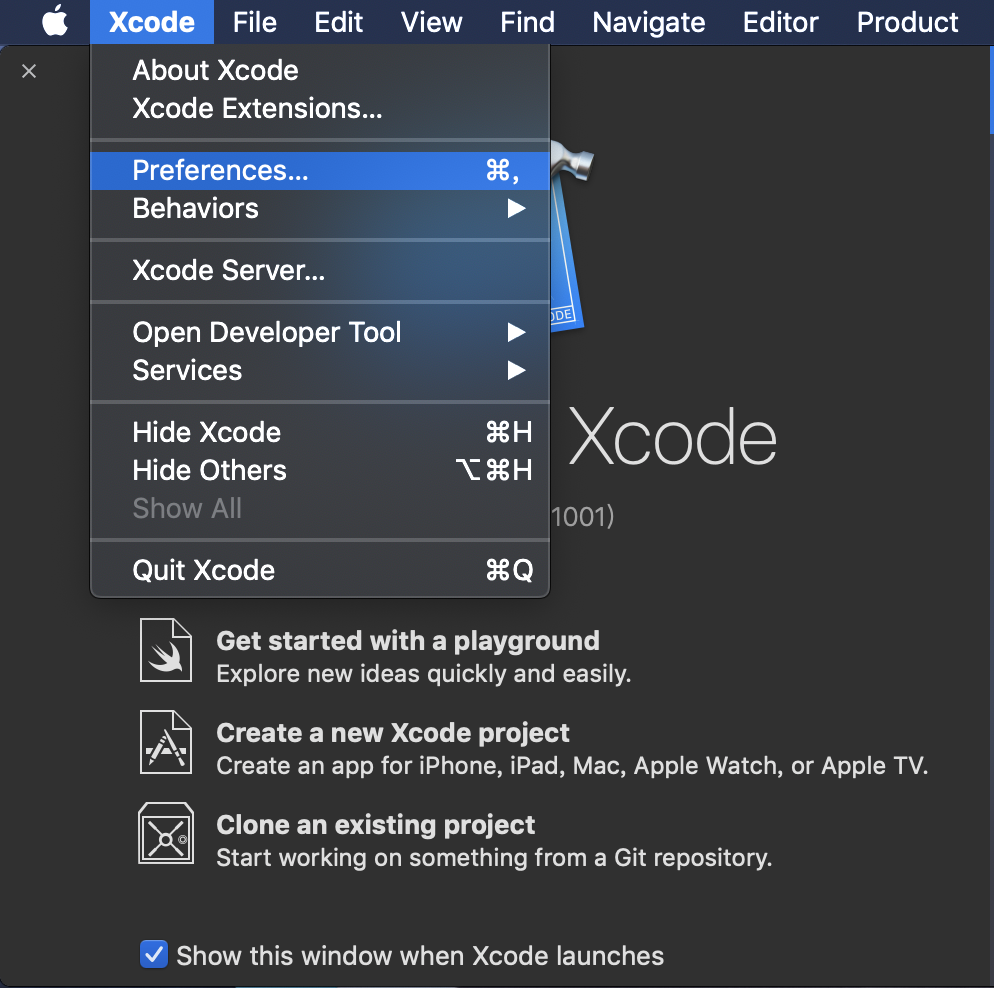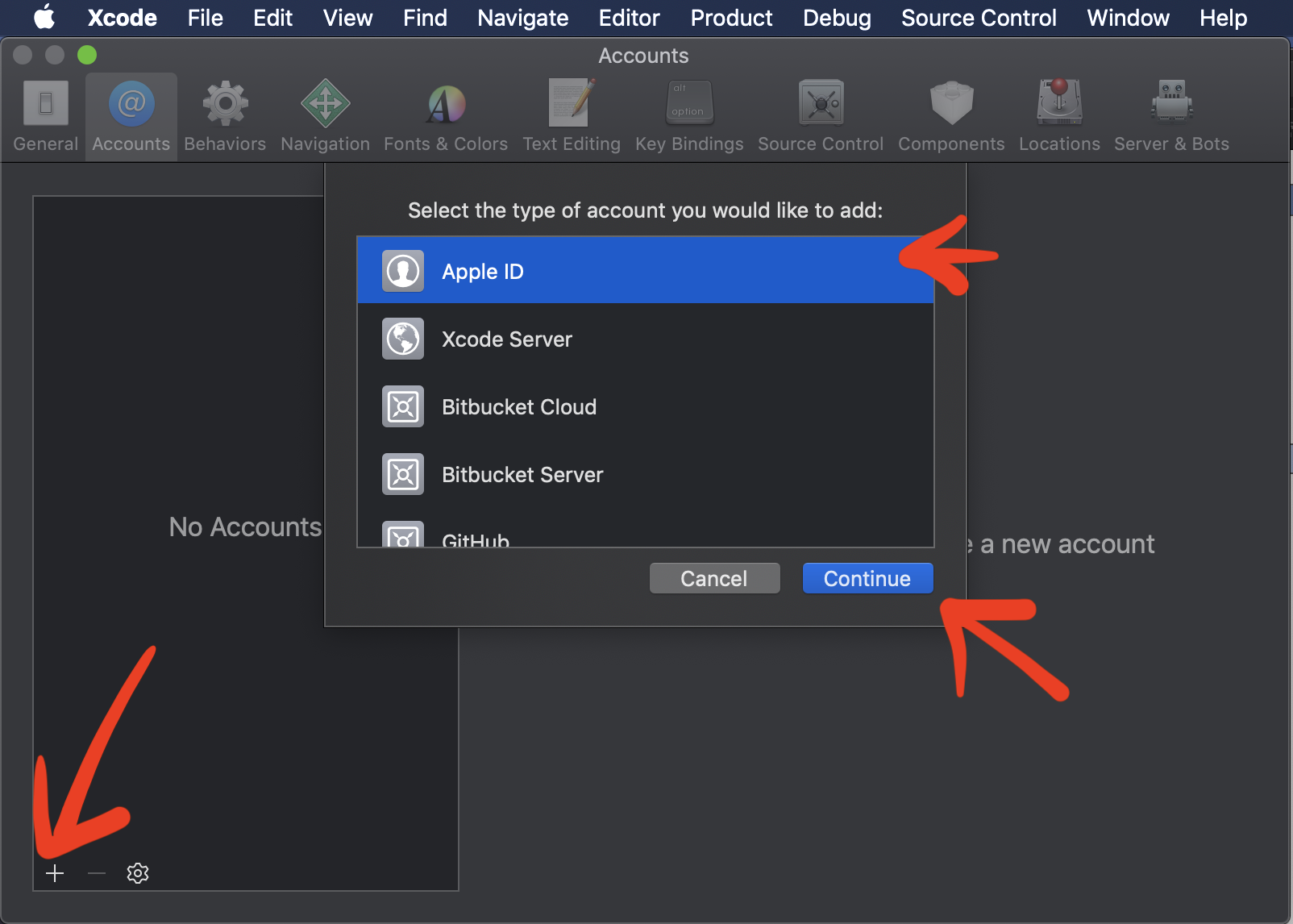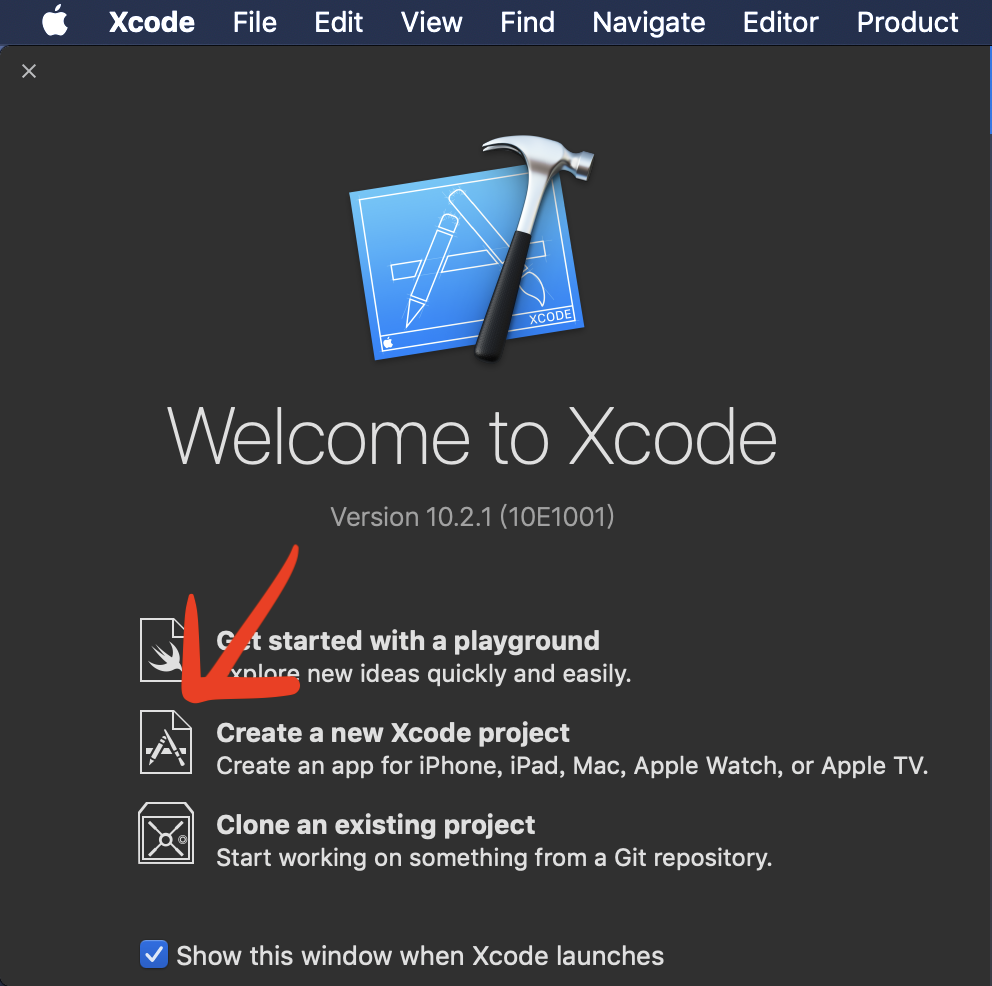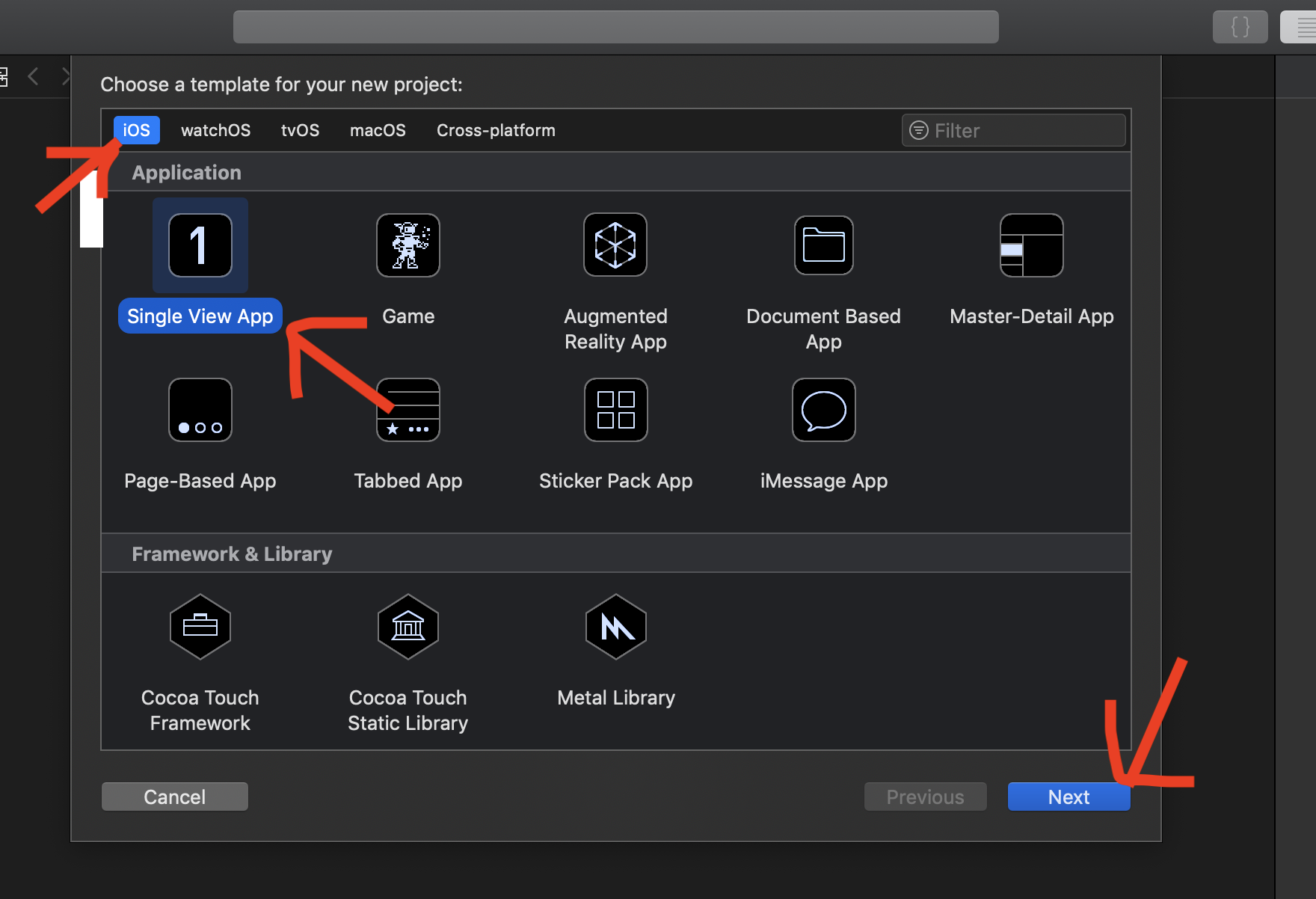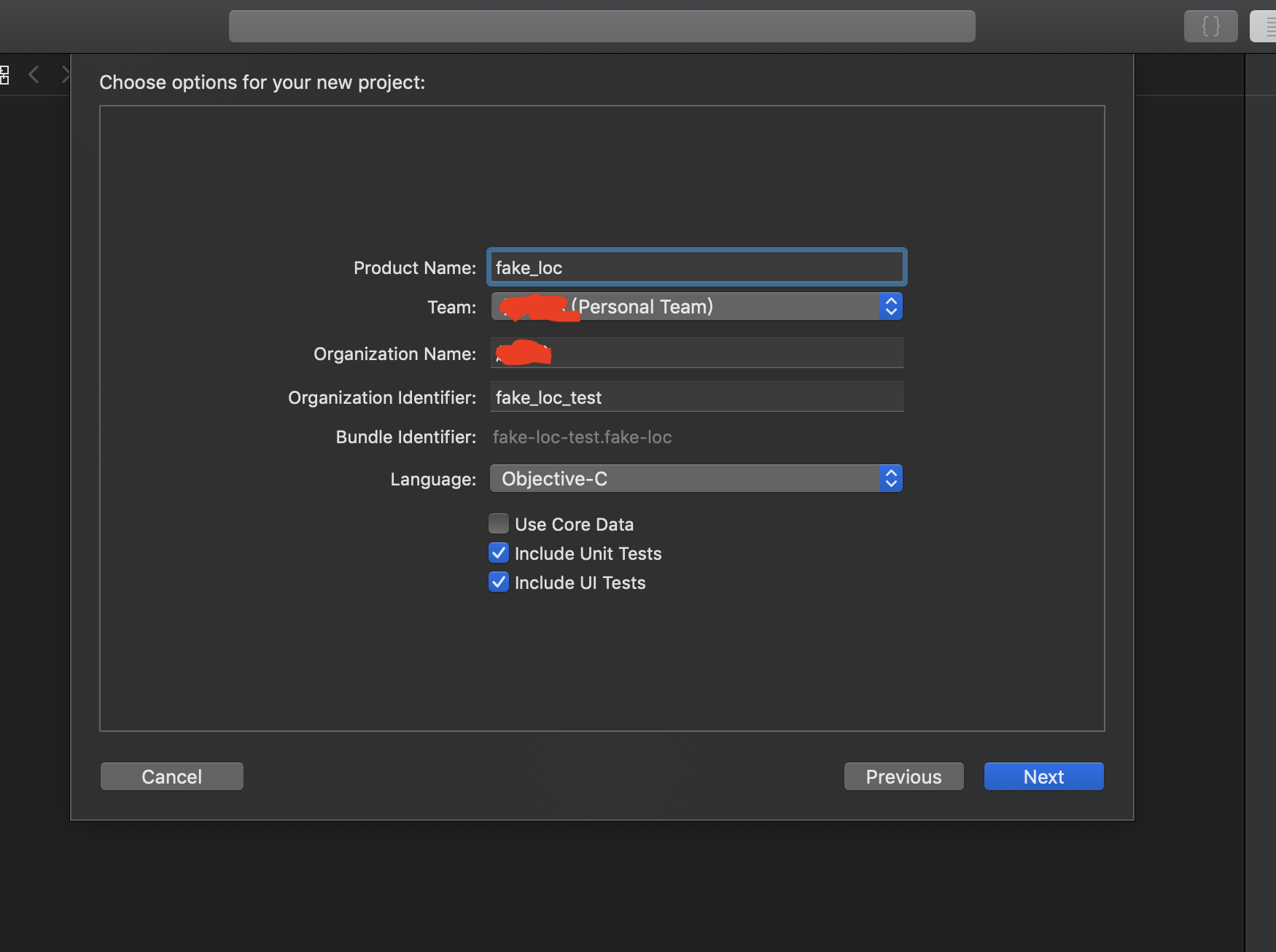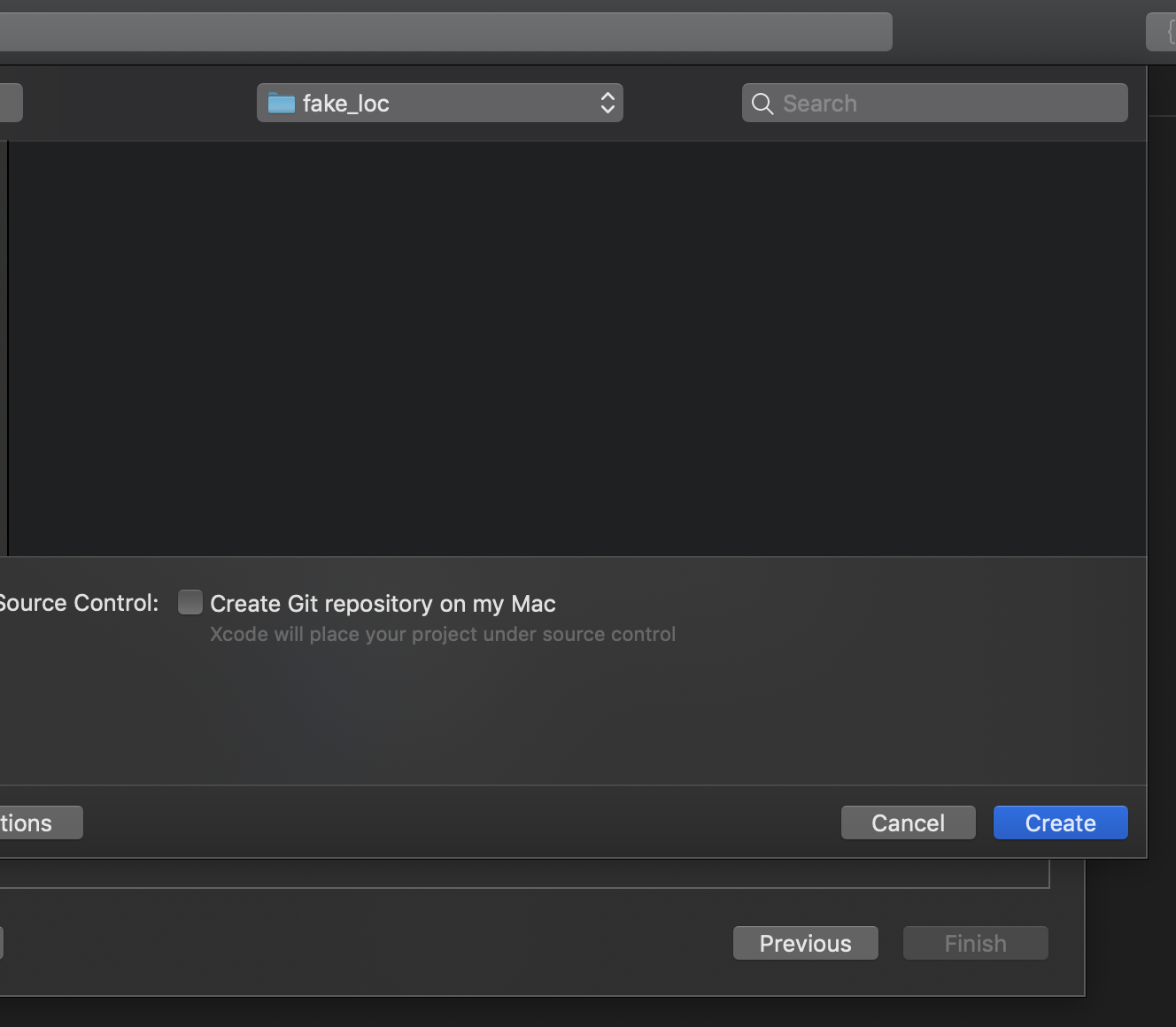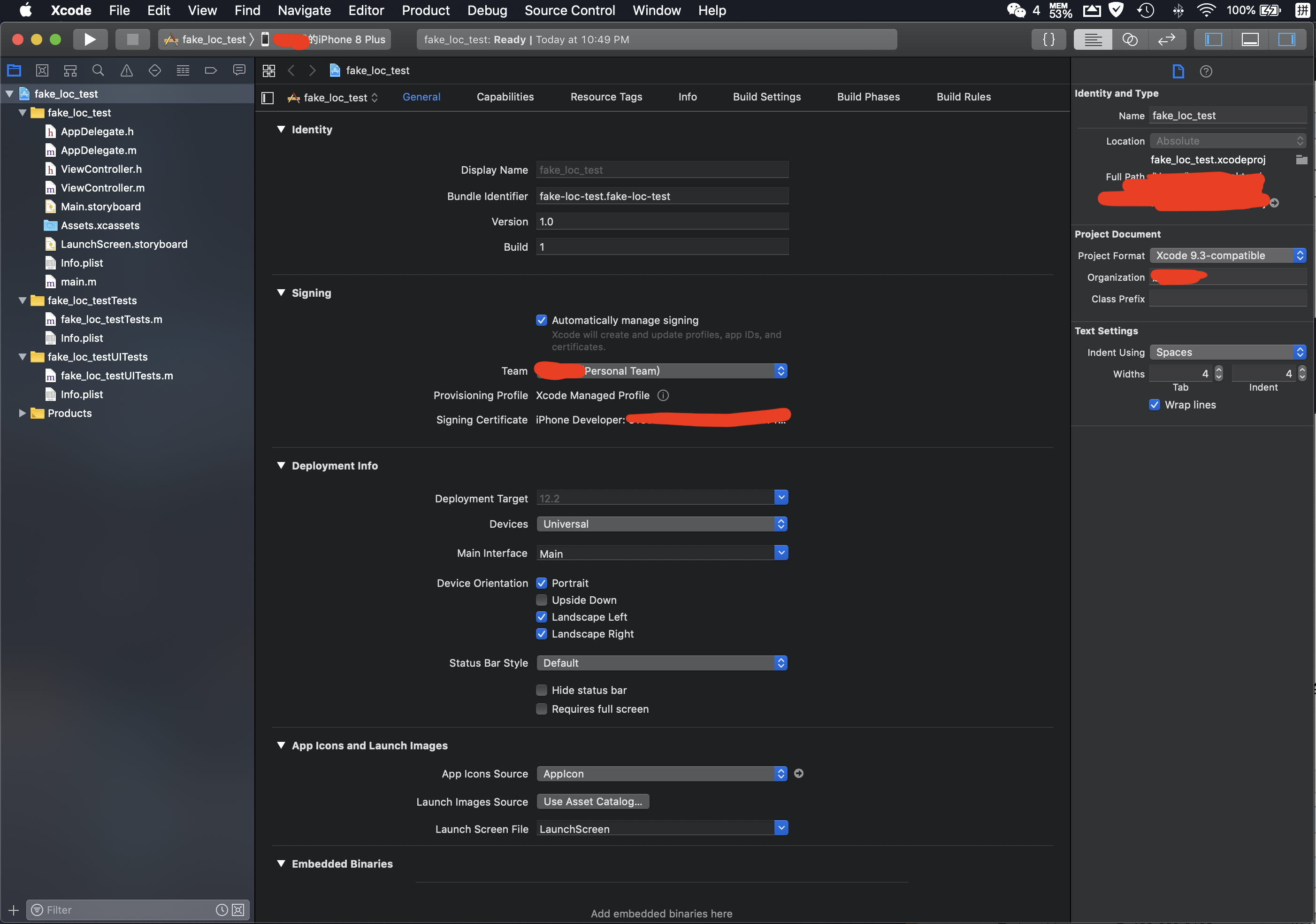## 2.使用Xcode创建一个含有gpx的文件

GPX（GPS eXchange Format，GPS交换格式）是一个XML格式，为应用软件设计的通用GPS数据格式，专门用来存储地理信息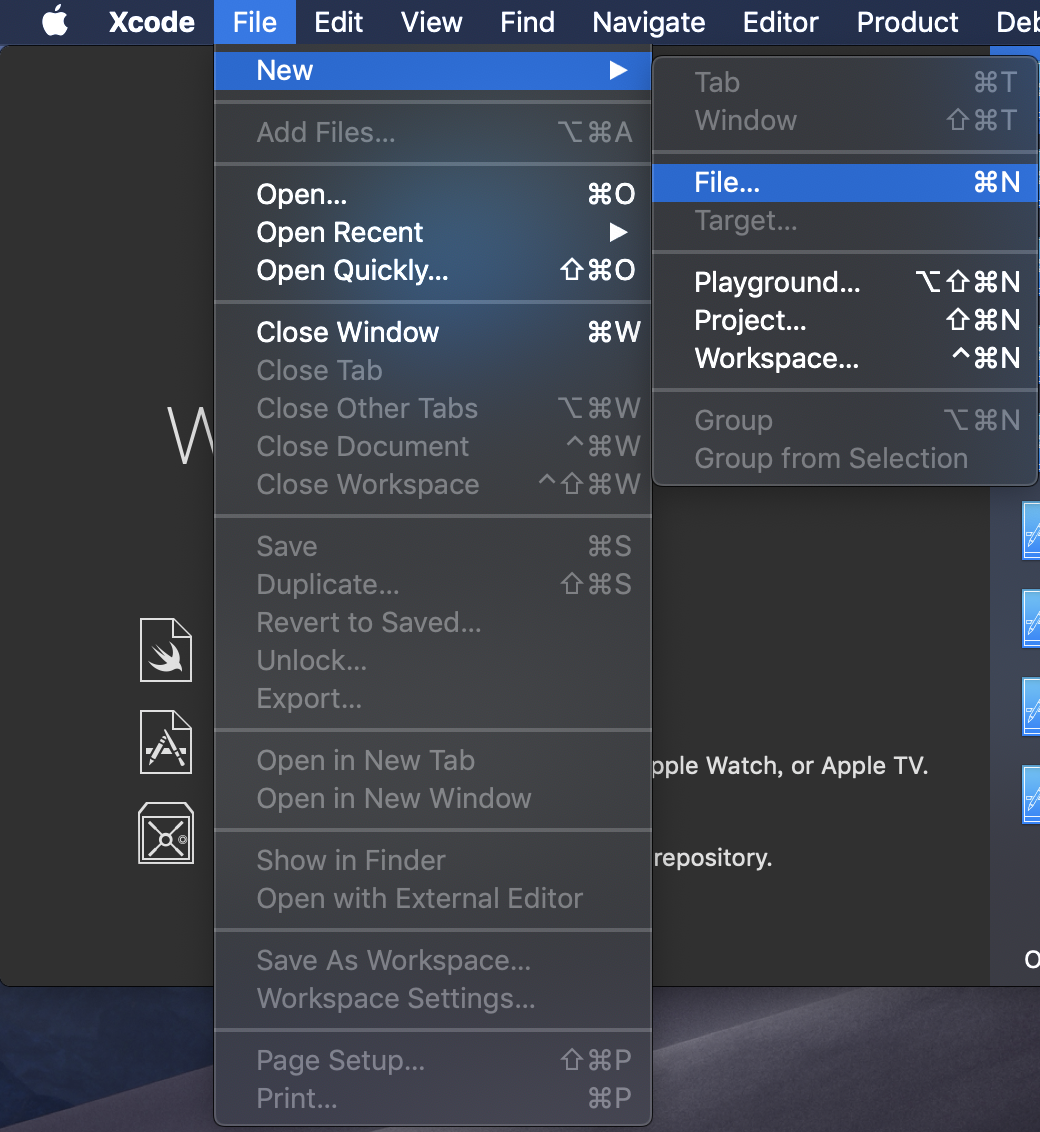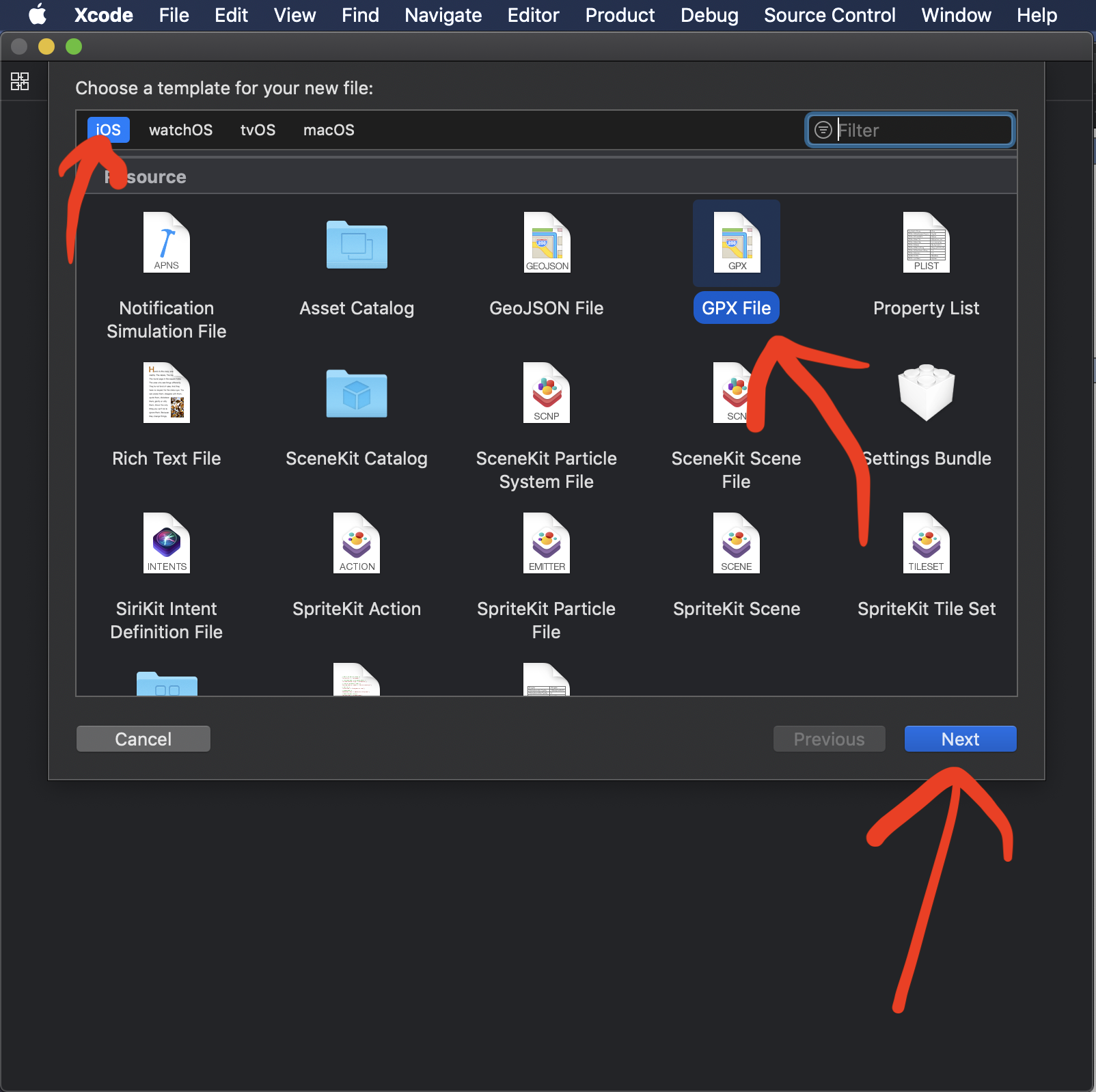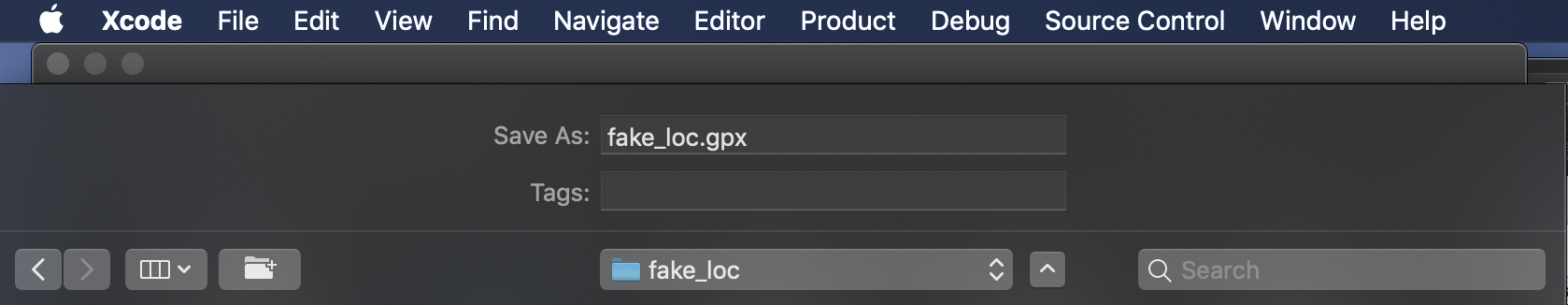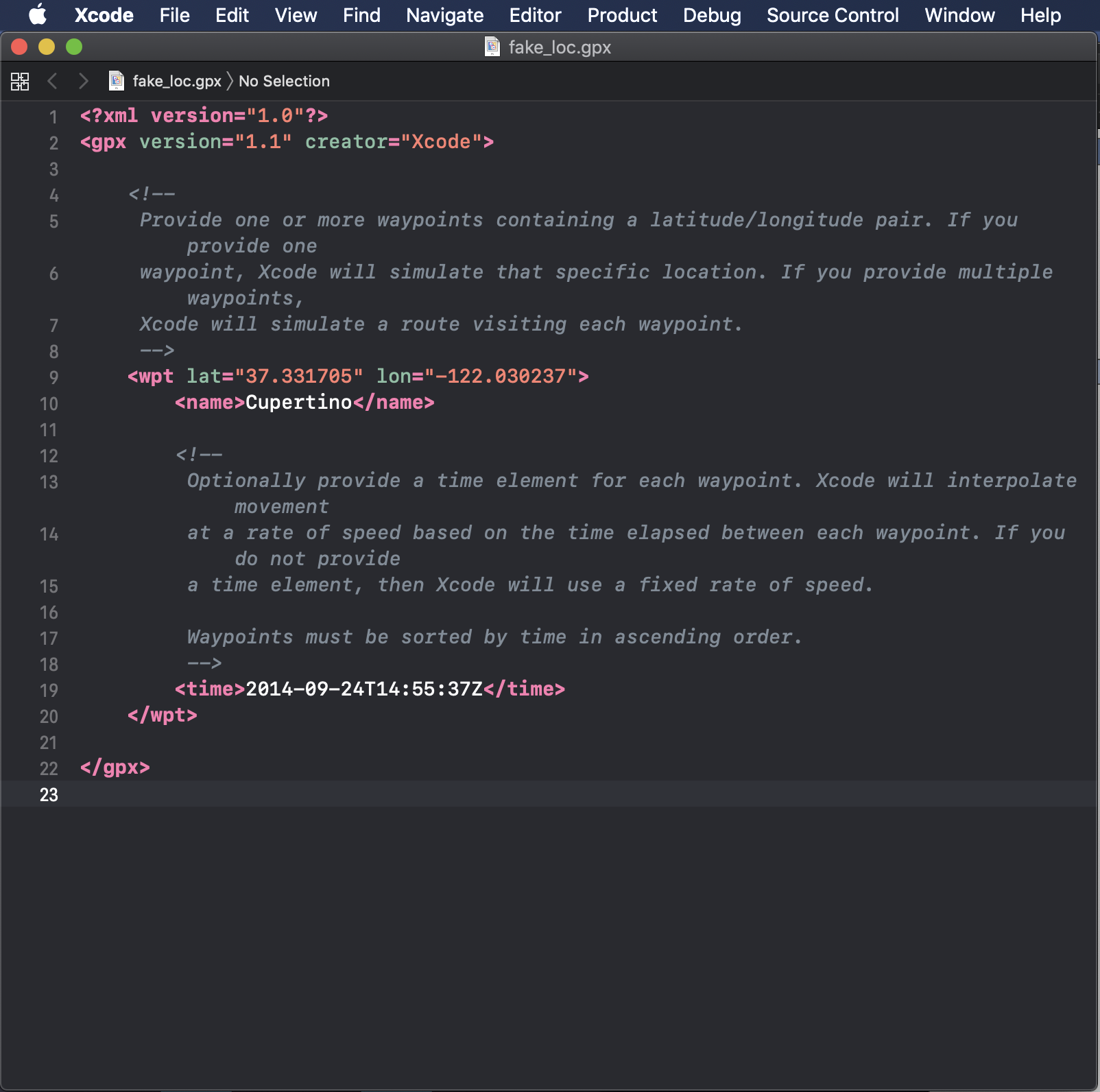“提供一个或多个包含纬度/经度对的点

如果提供一个点，Xcode将模拟那个特定的点

如果提供多个点，Xcode将依次模拟每一个点”

“可以选择为每一个坐标点添加一个时间标签可，Xcode可以根据时间标签的顺序依次移动到每一个坐标点，并根据时间点来插入速度X

如果不提供一个时间标签，那么Xcode将使用一个固定的速度

航路点必须按时间升序排序

## 3.使用Python来自动生成一系列轨迹点

mac下python与sublime text3的环境配置在第一篇随笔中有记录

`<?xml version="1.0"?><gpx version="1.1" creator="Xcode"> <wpt lat="坐标点经度1" lon="坐标点纬度1"> </wpt> <wpt lat="坐标点经度2" lon="坐标点纬度2"> </wpt> <wpt lat="坐标点经度3" lon="坐标点纬度3"> </wpt> #...... #以此类推</gpx>`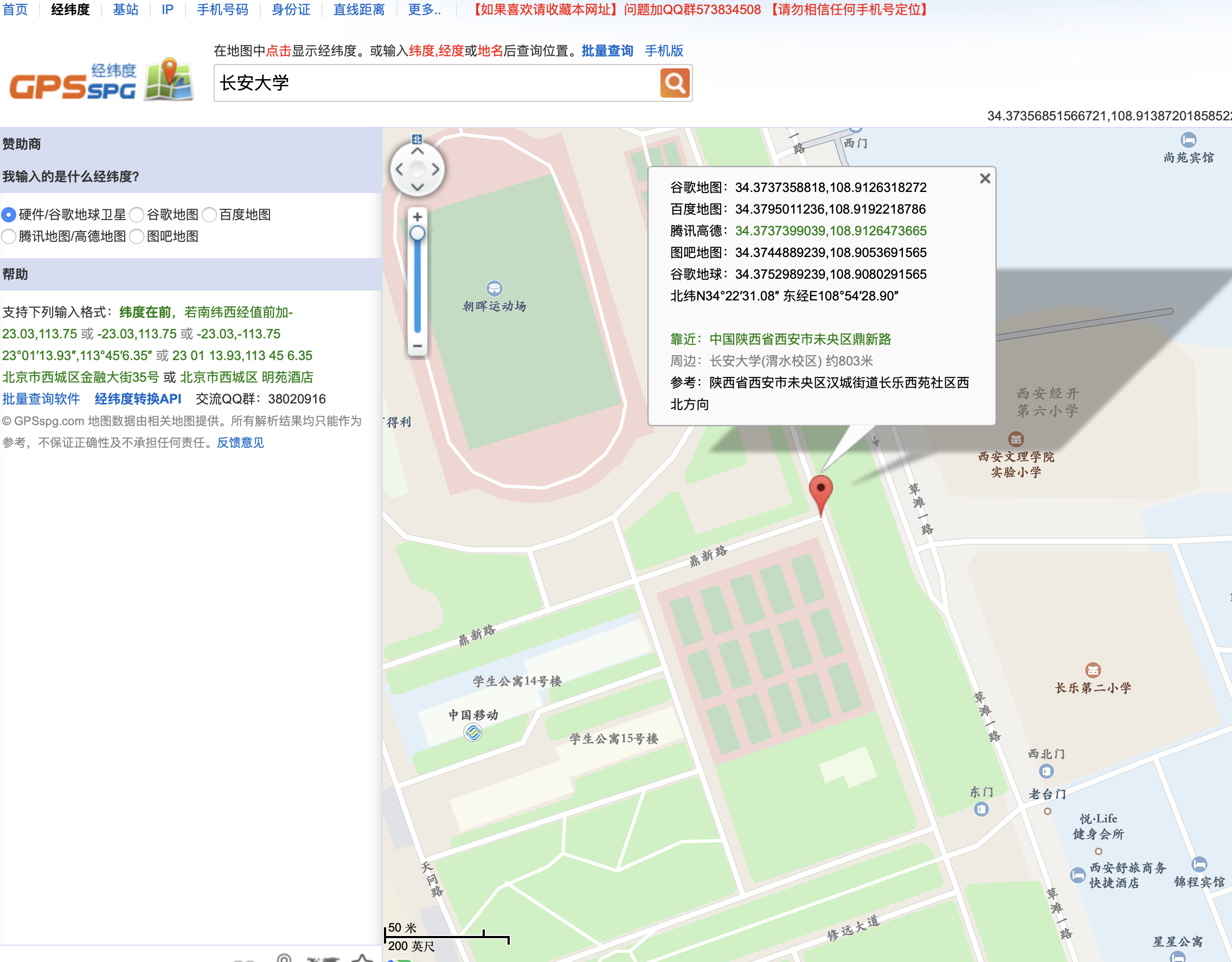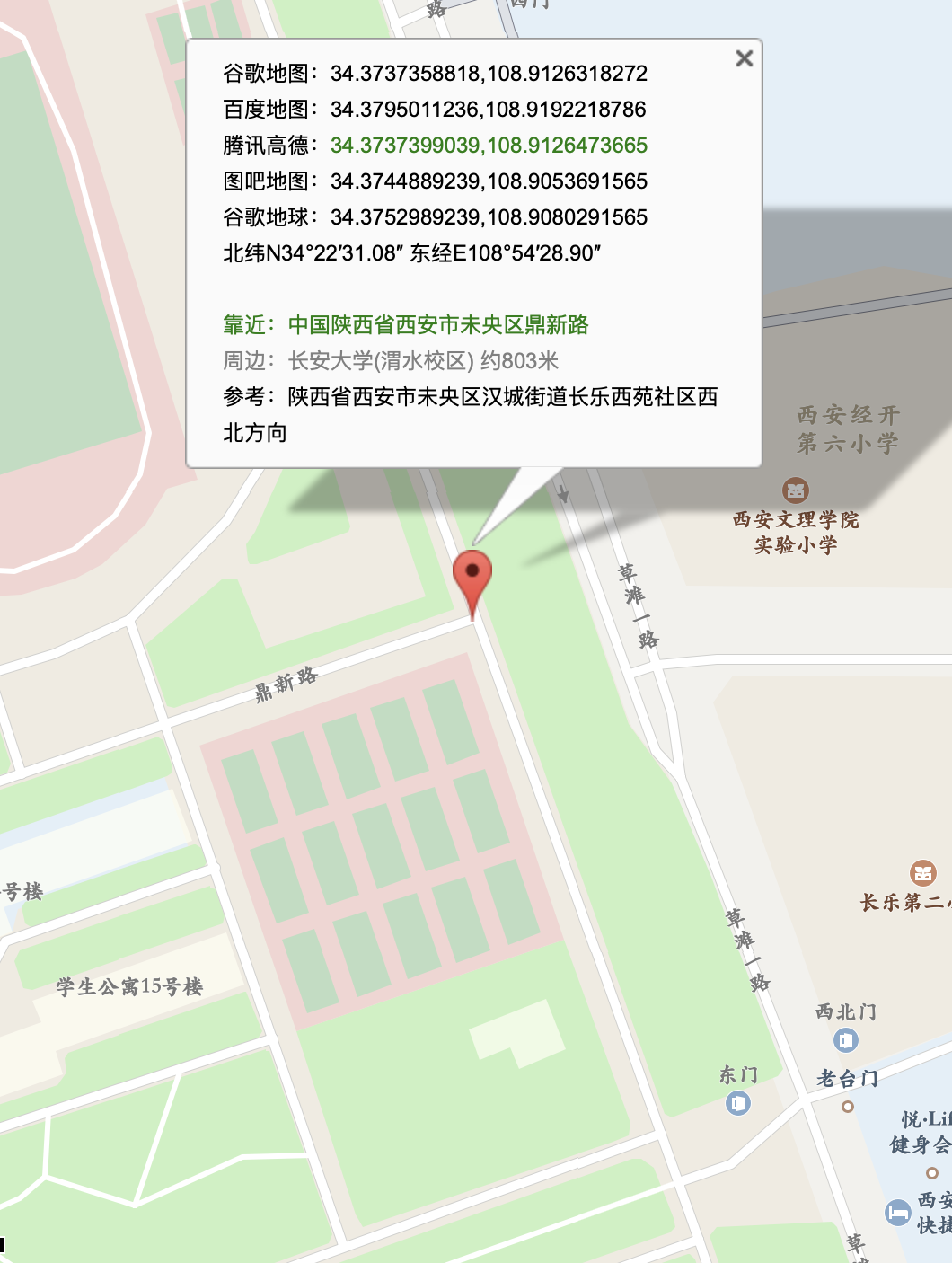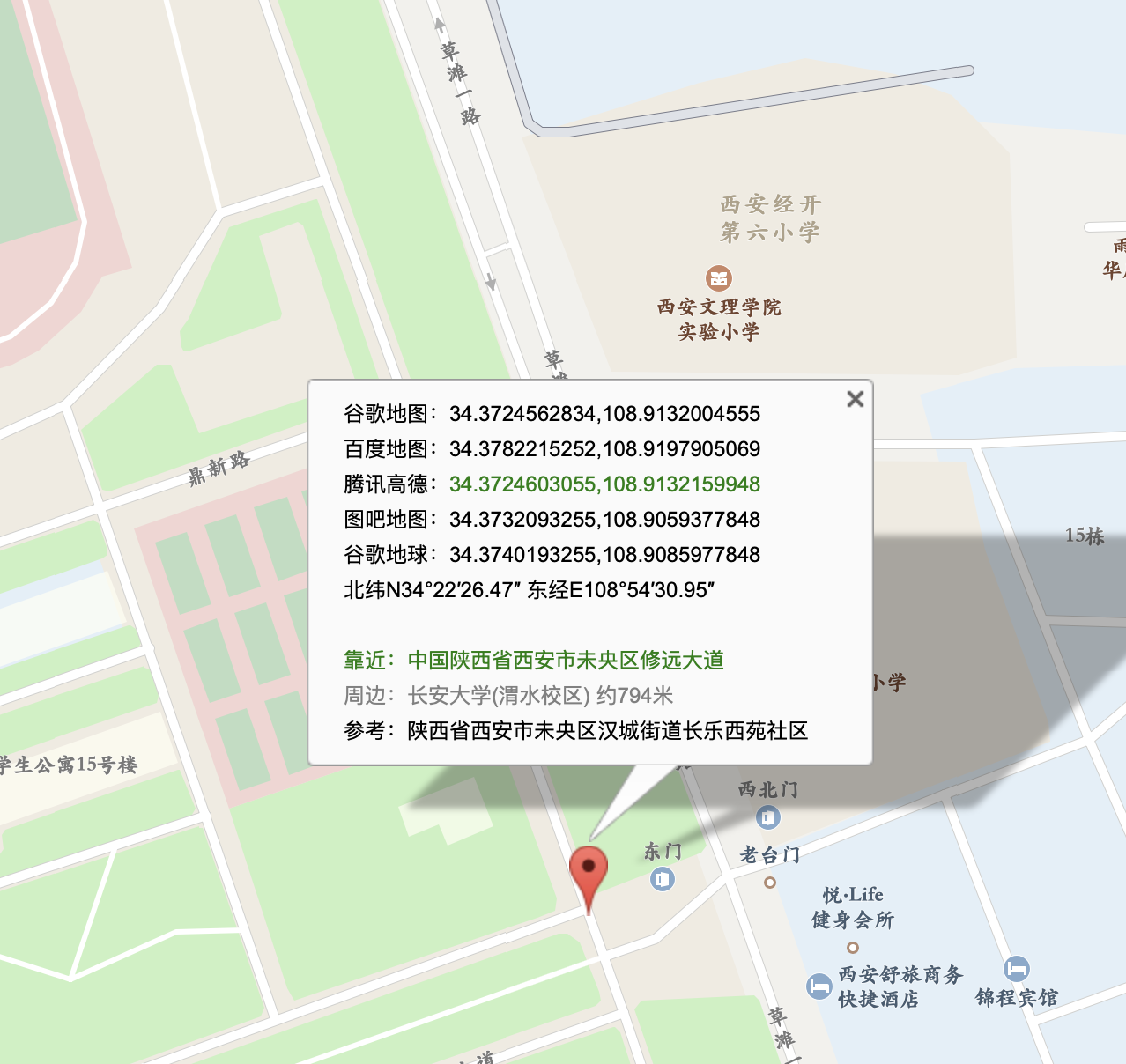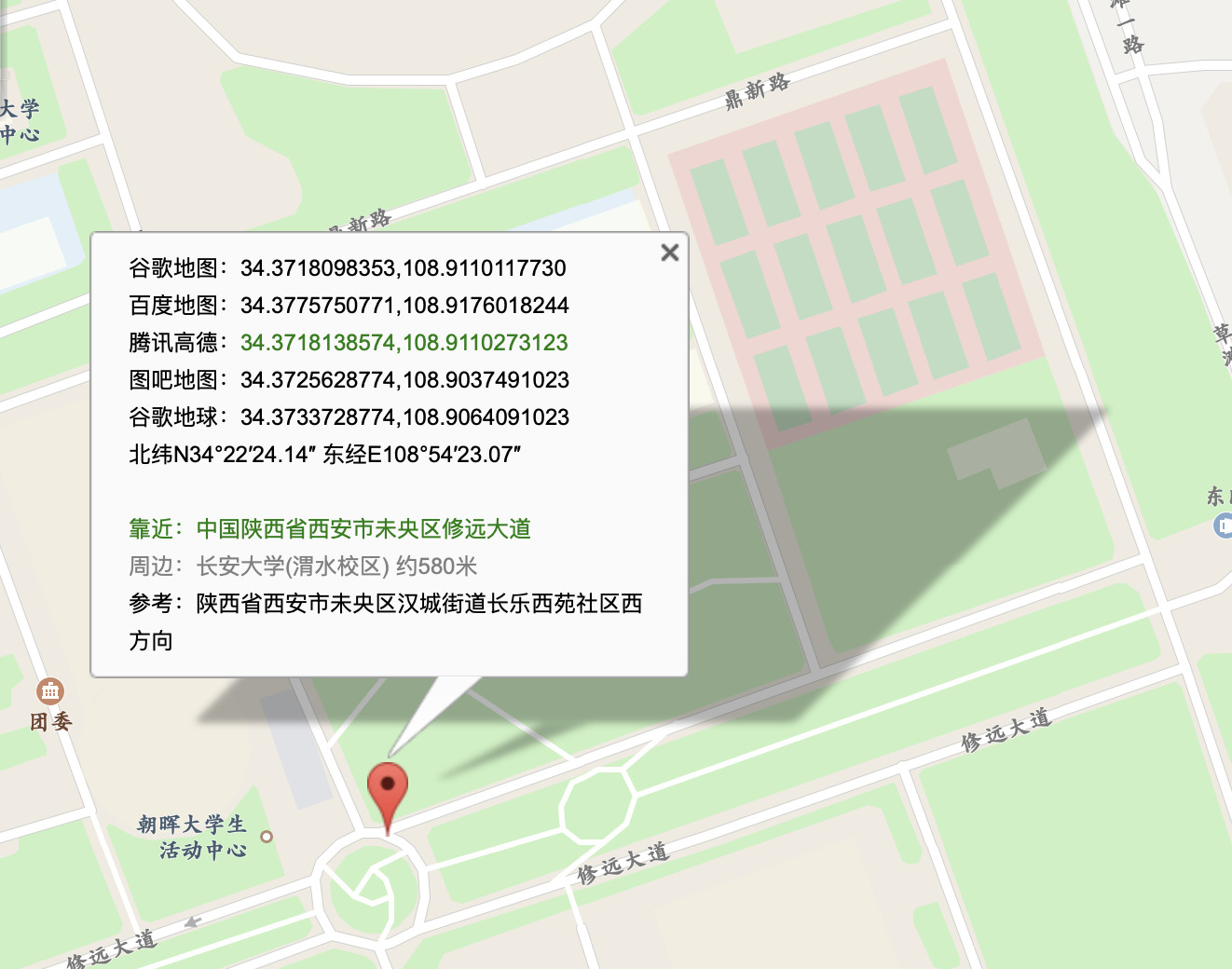lat：34.3752989239

lon：108.9080291565

lat：34.3740193255

lon：108.9085977848

lat：34.3733728774

lon：108.9064091023`import random#初始点信息a1 = 34.3752989239;b1 = 108.9080291565;#中间点信息a2 = 34.3740193255;b2 = 108.9085977848;for i in range( 1,1000 ): while( a1 >= a2 ):  # 循环条件 经度未到达中间点时执行  xa = round( random.uniform(0.2, 7) ) # 随机数，用于模拟人跑动  xb = round( random.uniform(12, 15) )  # 竖直移动  a1 = round( a1 - 0.0000072 * xa , 10 )  b1 = round( b10.0000008 * xb , 10 )  # 输出信息  print( '<wpt lat="'str( a1 )'"'' ''lon="'str( b1 )'">' )  print( '</wpt>' )for i in range( 1,1000 ): xa = round( random.uniform(2, 8) ) xb = round( random.uniform(1, 3) ) # 水平移动 a2 = round( a2 - 0.000003 * xa, 10 ) b2 = round( b2 - 0.000025 * xb, 10 ) print( '<wpt lat="`